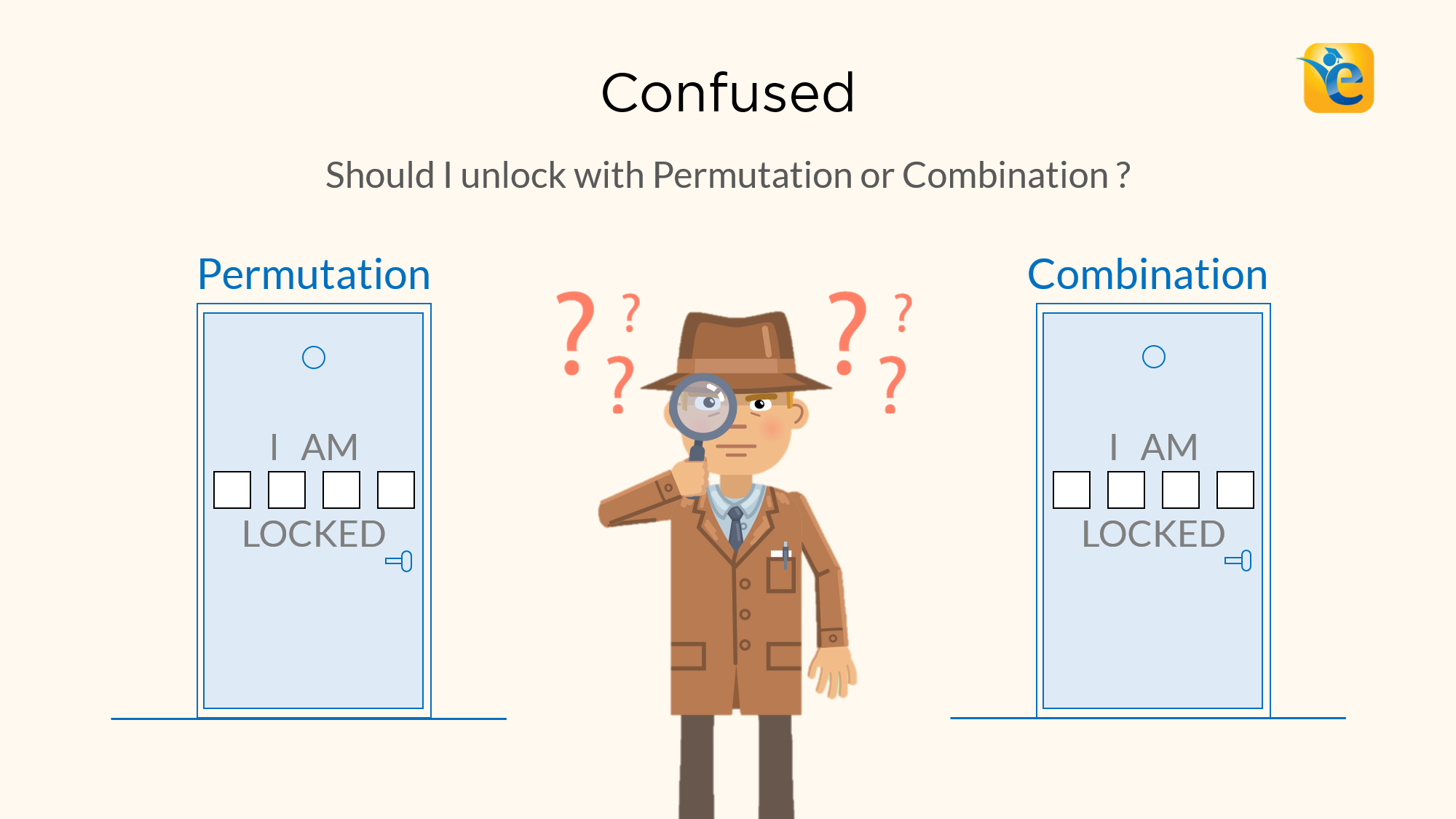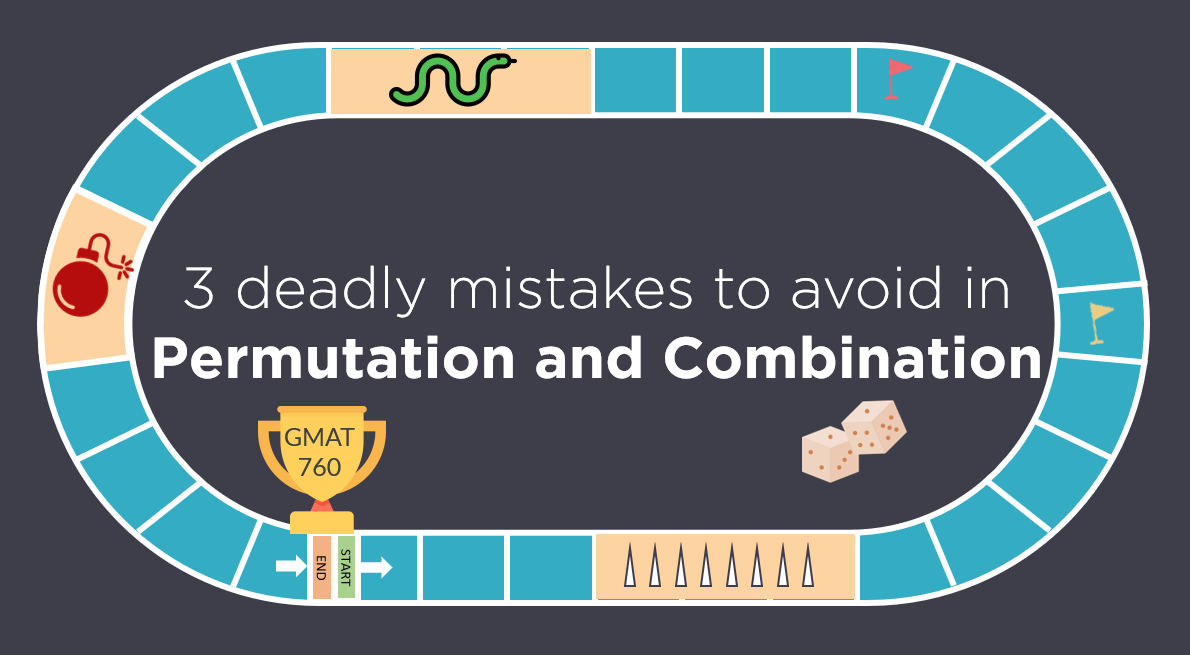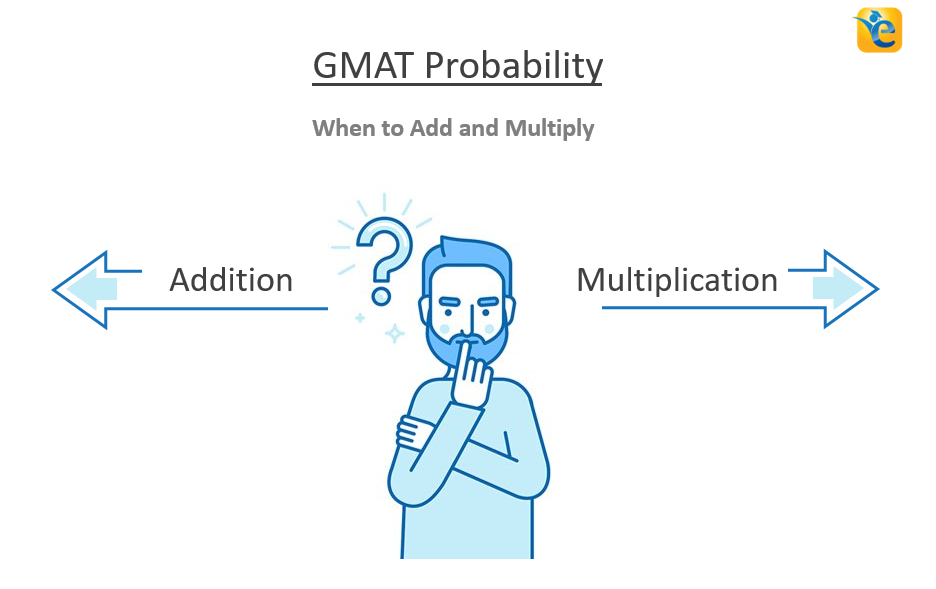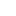Payal Tandon
Co-founder, e-GMAT
Welcome to e-GMAT Support!
I am Payal, Co-Founder of e-GMAT.
Feel free to ask any Query.We will be contacting you soon on# Difference between Permutation and Combination explained with examples

Permutations and Combinations is a topic full of conundrums. The biggest one is, understanding the difference between permutation and combination. Should I solve this...# Permutation and Combination | Avoid these 3 Mistakes | GMAT Quant

Like any GMAT Quant topic, Permutation and Combination has its own traps. Most students fall in these traps and ultimately, are not able to secure their target GMAT score....# GMAT Permutation and Combination | When to Add and Multiply

Are you always confused whether to add or multiply the cases while solving GMAT permutation and combination questions? Do you often get questions incorrect simply because...# GMAT Algebra and Algebraic Expressions | Linear & Quadratic Equations

The word Algebra was derived from the Arabic word “al-jabr” meaning “reunion of broken parts”.  In algebra, arithmetic computations are carried out...

# If x, y, and k are positive and x is less than y, then (x + k) / (y + k)  is – OGQR 2020 Question #80 with Solution

OGQR 2020: Question No. 80 If x, y, and k are positive and x is less than y, then (x + k) / (y + k) is SourceOGQR 2020TypeProblem...

# If half the result obtained when 2 is subtracted from 5x is equal to the sum of 10 and 3x, what is the value of x? – OGQR 2020 Question #77 with Solution

OGQR 2020: Question No. 77 If half the result obtained when 2 is subtracted from 5x is equal to the sum of 10 and 3x, what is the value...

# How old is Jane? – OGQR 2020 Question #208 with Solution

OGQR 2020: Question No. 208 How old is Jane? Ten years ago, she was one-third as old as she is now. In 15 years, she will be twice as...

# Last year Publisher X published 1,100 books, consisting of first editions, revised editions, and reprints. How many first editions did Publisher X publish last year? – OGQR 2020 Question #207 with Solution

OGQR 2020: Question No. 207 Last year Publisher X published 1,100 books, consisting of first editions, revised editions, and...

# More articles

## GMAT Algebra and Algebraic Expressions | Linear & Quadratic Equations

A 9 min read The word Algebra was derived from the Arabic word “al-jabr” meaning “reunion of...

## If x, y, and k are positive and x is less than y, then (x + k) / (y + k)  is – OGQR 2020 Question #80 with Solution

A < 1 min read OGQR 2020: Question No. 80 If x, y, and k are positive and x is less than y, then (x + k) / (y + k) is SourceOGQR 2020TypeProblem SolvingTopicAlgebraSub-TopicRatio / InequalityDifficultyMedium Solution Given In...

## If half the result obtained when 2 is subtracted from 5x is equal to the sum of 10 and 3x, what is the value of x? – OGQR 2020 Question #77 with Solution

A < 1 min read OGQR 2020: Question No. 77 If half the result obtained when 2 is subtracted from 5x is equal to the sum of 10 and 3x, what is the value of x? Source OGQR 2020 Type Problem Solving Topic Algebra Sub-Topic Linear...

## How old is Jane? – OGQR 2020 Question #208 with Solution

A < 1 min read OGQR 2020: Question No. 208 How old is Jane? Ten years ago, she was one-third as old as she is now. In 15 years, she will be twice as old as she is now. Source OGQR 2020 Type Data Sufficiency Topic Algebra...

## Last year Publisher X published 1,100 books, consisting of first editions, revised editions, and reprints. How many first editions did Publisher X publish last year? – OGQR 2020 Question #207 with Solution

A 2 min read OGQR 2020: Question No. 207 Last year Publisher X published 1,100 books, consisting of first editions, revised editions, and reprints. How many first editions did Publisher X publish last year? The...

## GMAT Quant OG 2020 Question #312 with Solution – “In any sequence of n nonzero numbers…”

A 2 min read PQID: DS57502.01 | OG 2020: Question No. 312 In any sequence of n nonzero numbers, a pair of consecutive terms with opposite signs represents a sign change. For example, the sequence –2, 3, –4, 5 has three sign...

## If a and b are integers, and b > 0, does a-1 /b+1 = a/b? – OG 2020 Question #297 with Solution

A < 1 min read OG 2020: Question No. 297 If a and b are integers, and b > 0, does a-1 /b+1 = a/b? a = b − 4 a = –b Source OG 2020 Type Data Sufficiency Topic Algebra Sub-Topic Linear Equations Difficulty Easy –...

## Is x = y? – OG 2020 Question #373 with Solution

A < 1 min read OG 2020: Question No. 373 Is x = y? 2x/3 – y/3 = 1/3 x/ 4 – y/4 = 0 Source OG 2020 Type Data Sufficiency Topic Algebra Sub-Topic Simple Algebric Expression Difficulty Easy – Medium Solution...

## Is the average (arithmetic mean) of the numbers x, y, and z greater than z? – OG 2020 Question #320 with Solution

A 2 min read OG 2020: Question No. 320 Is the average (arithmetic mean) of the numbers x, y, and z greater than z? z − x < y − z x < z < y Source OG 2020 Type Data Sufficiency Topic Algebra, Number Properties Sub-Topic...

## Jack picked 76 apples. Of these, he sold 4y apples to Juanita and 3t apples to Sylvia. If he kept the remaining apples, how many apples did he keep? (t and y are positive integers.) – OG 2020 Question #313 with Solution

A 2 min read OG 2020: Question No. 313 Jack picked 76 apples. Of these, he sold 4y apples to Juanita and 3t apples to Sylvia. If he kept the remaining apples, how many apples did he keep? (t and y are positive integers.) y ≥ 15...###### Browse Related Topics# Achieve 740+on the GMAT in 30 days!Sign up for our free trial and get:400+ Practice questions with detailed solutions10+ hours of AI-driven video lessonsAdaptive mock test with ESR+ analysis